Syntax Directed Translation

# Syntax Directed Translation - Notes | Study Compiler Design - Computer Science Engineering (CSE)

 1 Crore+ students have signed up on EduRev. Have you?

3.Syntax Directed Translation:
· A formalist called as syntax directed definition is used fort specifying translations for programming language constructs.
· A syntax directed definition is a generalization of a context free grammar in which each grammar symbol has associated set of attributes and each and each productions is associated with a set of semantic rules

Definition of (syntax Directed definition ) SDD :

SDD is a generalization of CFG in which each grammar productions X->α is associated with it a set of semantic rules of the form

a: = f(b1,b2…..bk)

Where a is an attributes obtained from the function f.

• A syntax-directed definition is a generalization of a context-free grammar in which:

– Each grammar symbol is associated with a set of attributes.

– This set of attributes for a grammar symbol is partitioned into two subsets called synthesized and inherited attributes of that grammar symbol.

– Each production rule is associated with a set of semantic rules.

• Semantic rules set up dependencies between attributes which can be represented by a dependency graph.

• This dependency graph determines the evaluation order of these semantic rules.

• Evaluation of a semantic rule defines the value of an attribute. But a semantic rule may also have some side effects such as printing a value.

The two attributes for non terminal are :

1) Synthesized attribute (S-attribute) : (↑)

An attribute is said to be synthesized attribute if its value at a parse tree node is determined from attribute values at the children of the node 2) Inherited attribute: (↑,→)

2) Inherited attribute: (↑,→)

An inherited attributeis one whose value at parse tree node is determined in terms of attributes at the parent and | or siblings of that node.

• The attribute can be string, a number, a type, a, memory location or anything else.
• The parse tree showing the value of attributes at each node is called an annotated parse tree.

The process of computing the attribute values at the node is called annotating or decorating the parse tree.Terminals can have synthesized attributes, but not inherited attributes.

Annotated Parse Tree

• A parse tree showing the values of attributes at each node is called an Annotated parse tree.

• The process of computing the attributes values at the nodes is called annotating (or decorating) of the parse tree.

• Of course, the order of these computations depends on the dependency graph induced by the semantic rules. Ex1:1) Synthesized Attributes :

Ex: Consider the CFG : S→ EN E→ E+T E→E-T E→ T T→ T*F T→T/F T→F F→ (E) F→digit N→;

Solution: The syntax directed definition can be written for the above grammar by using semantic actions for each production.

Production rule            Semantic actions
S →EN                        S.val=E.val
E →E1+T                     E.val =E1.val + T.val
E →E1-T                      E.val = E1.val – T.val
E →T                           E.val =T.val
T →T*F                       T.val = T.val * F.val
T →T|F                        T.val =T.val | F.val
F → (E)                        F.val =E.val
T →F                           T.val =F.val
F →digit                       F.val =digit.lexval
N →;                            can be ignored by lexical Analyzer as;
I is terminating symbol

For the Non-terminals E,T and F the values can be obtained using the attribute “Val”.

The taken digit has synthesized attribute “lexval”.

In S→EN, symbol S is the start symbol. This rule is to print the final answer of expressed.

Following steps are followed to Compute S attributed definition

1. Write the SDD using the appropriate semantic actions for corresponding production rule of the given Grammar.

2. The annotated parse tree is generated and attribute values are computed. The Computation is done in bottom up manner.

3. The value obtained at the node is supposed to be final output.

PROBLEM 1:

Consider the string 5*6+7; Construct Syntax tree, parse tree and annotated tree.
Solution:

The corresponding annotated parse tree is shown below for the string 5*6+7;

Syntax tree: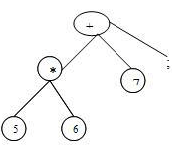Annotated parse tree :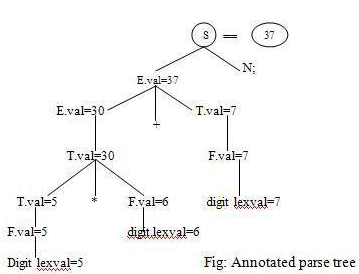SDDs are more readable and hence useful for specifications Disadvantages: not very efficient.
Ex2:

PROBLEM :
Consider the grammar that is used for Simple desk calculator. Obtain the
Semantic action and also the annotated parse tree for the string

3*5+4n. L→En E→E1+T
E→T
T→T1*F
T→F
F→ (E)
F→digit

Solution :

Production rule Semantic actions

L→En                                L.val=E.val
E→E1+T                          E.val=E1.val + T.val
E→T                                E.val=T.val
T→T1*                             F T.val=T1.val*F.val
T→F                                T.val=F.val
F→(E)                             F.val=E.val
F→digit                          F.val=digit.lexval

The corresponding annotated parse tree U shown below, for the string 3*5+4n.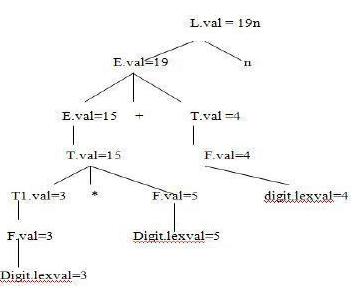Dependency Graphs: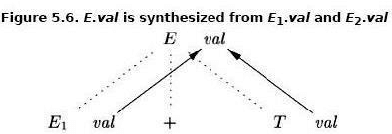Dependency graph and topological sort:

• For each parse-tree node, say a node labeled by grammar symbol X, the dependency graph has a node for each attribute associated with X.
•  If a semantic rule associated with a production p defines the value of synthesized attribute A.b in terms of the value of X.c. Then the dependency graph has an edge from X.c to A.b
• If a semantic rule associated with a production p defines the value of inherited attribute B.c in terms of the value X.a. Then , the dependency graph has an edge from X.a to B.c.

Applications of Syntax-Directed Translation
• Construction of syntax Trees – The nodes of the syntax tree are represented by objects with a suitable number of fields. – Each object will have an op field that is the label of the node. – The objects will have additional fields as follows
• If the node is a leaf, an additional field holds the lexical value for the leaf. A constructor function Leaf (op, val) creates a leaf object.
• If nodes are viewed as records, the Leaf returns a pointer to a new record for a leaf.
• If the node is an interior node, there are as many additional fields as the node has children in the syntax tree. A constructor function Node takes two or more arguments: Node (op , c1,c2,…..ck) creates an object with first field op and k additional fields for the k children c1,c2,…..ck

Syntax-Directed Translation Schemes
A SDT scheme is a context-free grammar with program fragments embedded within production bodies .The program fragments are called semantic actions and can appear at any position within the production body.

Any SDT can be implemented by first building a parse tree and then pre-forming the actions in a leftto-right depth first order. i.e during preorder traversal.
The use of SDT’s to implement two important classes of SDD’s
1. If the grammar is LR parsable, then SDD is S-attributed.
2. If the grammar is LL parsable, then SDD is L-attributed.

Postfix Translation Schemes The postfix SDT implements the desk calculator SDD with one change: the action for the first produ  ction prints the value. As the grammar is LR, and the SDD is S-attributed.
L →E n {print(E.val);}
E → E1 + T { E.val = E1.val + T.val }
E → E1 - T { E.val = E1.val - T.val }
E → T { E.val = T.val }
T → T1 * F { T.val = T1.val * F.val }
T → F { T.val = F.val }
F → ( E ) { F.val = E.val }
F → digit { F.val = digit.lexval }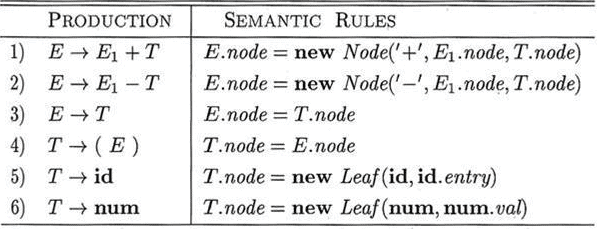The document Syntax Directed Translation - Notes | Study Compiler Design - Computer Science Engineering (CSE) is a part of the Computer Science Engineering (CSE) Course Compiler Design.
All you need of Computer Science Engineering (CSE) at this link: Computer Science Engineering (CSE)

## Compiler Design

15 videos|55 docs|30 tests
 Use Code STAYHOME200 and get INR 200 additional OFF

## Compiler Design

15 videos|55 docs|30 tests

Track your progress, build streaks, highlight & save important lessons and more!

,

,

,

,

,

,

,

,

,

,

,

,

,

,

,

,

,

,

,

,

,

;